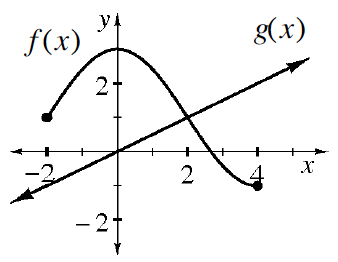### Home > CCAA8 > Chapter 11 Unit 12 > Lesson CCA: 11.1.1 > Problem11-12

11-12.

Examine the graphs of relations $f\left(x\right)$ and $g\left(x\right)$ below. Use the graph to approximate the values below (if possible).1. $f\left(0\right)$

When $x$ is $0$ in the relation $f\left(x\right)$, what is $y$?

$3$

1. $g\left(4\right)$

When $x$ is $4$ in the relation $g\left(x\right)$, what is $y$?

$2$

1. $f\left(5\right)$

Is there a $y$-value for $f\left(x\right)$ when $x$ is $5$?

1. $g\left(0\right)$

See the hint for part (b).

1. $g\left(2\right)$

See the hint for part (b).

1. $f\left(2\right)$

See the hint for part (a).